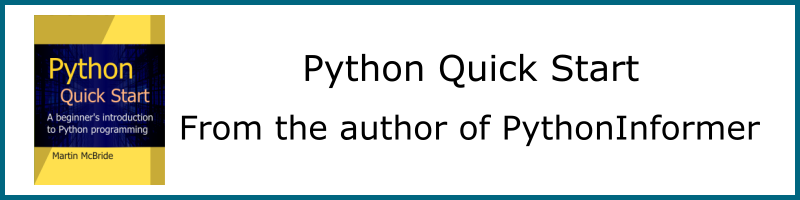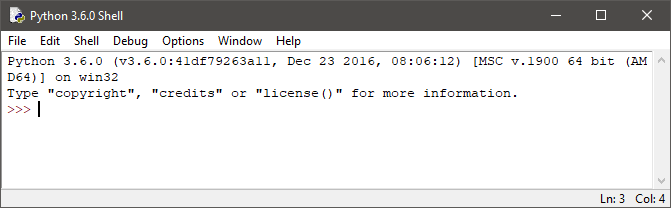# Using Python as a calculator

Martin McBride, 2018-07-22
Tags idle integer floating point conversion floor divide
Categories python language beginning pythonIn this lesson, we will use the IDLE program to interact with Python directly. This is a good way to get to know some of the basic things Python can do before we move on to do some real programming.

• Simple maths in Python
• Variables
• Strings

## Running IDLE

You should already have Python installed on your computer. If not, follow the instructions in the Introduction lesson. We recommend version 3.6 or higher.

Python comes with a simple, built-in user interface called IDLE. You should have already seen this in the first lesson.

Search for the IDLE program in the program finder (eg Start Menu in Windows, Dash in Ubuntu). Make sure that you have chosen V3.6. Here is the window that should appear. It is called the IDLE console window:The version of Python is displayed in the title bar and the initial message on the screen.

You can type instructions into this window. We will show this in bold, like this:

>>> 3 + 2

This means that you should type 3 + 2 into the window, and hit return. Don't type in the >>>, that is just the prompt Python displays! We will show Python's response in italics, like this:

5

So, a complete transaction might look like this:

>>> 10 * 3
30


Here, you have typed in 10 * 3 (10 multiplied by 3) followed by a return, and Python has replied with 30.

## Simple maths in Python

As you might have guessed, when you type in something like 3 + 2 in the IDLE console window, Python evaluates it and displays the answer, 5. Here are some more examples. In each case, type in the bold text and you should see Python print the answer (shown in italics). Try this:

>>> 1 + 2 + 4
7
>>> 1 + 2 - 4
-1
>>> 1 - 2 + 4
3


These are some simple additions and subtractions. Nothing too complicated here.

You can also multiply and divide. Try this:

>>> 3 * 5
15
>>> 3 / 2
1.5


Python uses * for multiply and / for divide, similar to most other programming languages (and spreadsheets).

By default, multiply and divide take precedence over add and subtract. You can use brackets to change this, just like in regular maths. Try this:

>>> 10 + 2 * 3
16
>>> (10 + 2) * 3
36


### Modulo

The modulo operator (represented by %) finds the remainder when one number is divided by another:

>>> 10 % 3
1
>>> 10 % 5
0


In this case, 10 divided by 3 is 3 remainder 1, so the modulo is 1. In the second case, 10 divided by 5 is 2 with no remainder, so the modulo is 0.

### Power

The power operator (represented by **) raises one number to the power of another. For example:

>> 5 ** 3
225
>>> 2 ** 4
16


In the first case, 5 to the power 3 is 5 * 5 * 5, or 225. In the second case, 2 to the power 4 is 2 * 2 * 2 * 2, or 16.

These are all the basic maths operations you will need to know to start programming in Python.

## Integers and floating-point numbers

Python uses two different types of numbers:

• Integer numbers (called ints) are whole numbers like 1, 26, -2 or 0.
• Floating-point numbers (called floats) are numbers with a decimal point like 1.3, 17.8, -0.5, 6.0. Notice that even if the value is a whole number (like 6.0) the type of number is still a float.

We won't cover this in a lot of detail here, but the general rule is that you should use an int in any situation where the number really must be a whole number. Typically this includes numbers used to count or order things. For example, if we say that June is the 6th month of the year, and has 30 days, then 6 and 30 should be ints.

Floats are used for quantities that could have a fractional part, for example, the temperature is 18.5 degrees. Typically, physical quantities like length, speed, mass are floats.

### Conversion

In most cases, if all the numbers in a calculation are ints (no decimal point) the result will be an int. If some or all of the numbers are floats, the result will be a float. For example:

>>> 3 + 5
8
>>> 3.1 + 5
8.1


The main exception is division. When you divide two numbers, the result is always a float. Even if the result is a whole number, it is still a number of type float:

>>> 6 / 4
1.5
>>> 6 / 3
2.0


### Floor divide

If you divide two ints using the floor divide operator (represented by //) you will get an int result. The result will be rounded towards zero:

>>> 6 // 4
1
>>> 6 // 3
2


## Variables

A variable is a way to store (ie remember) a value to use later in your program. Try this:

>>> a = 3


This creates a variable called a and gives it a value 3. Python doesn't print a response to this, it just stores the value. Now try this:

>>> b = 6


This creates another variable called b and gives it a value 6.

You can find the value of a variable by typing its name:

>>> a
3
>>> b
6


### Calculations with variables

You can use a variable in a calculation, instead of a value. The current value of the variable will be used:

>>> a + 2
5
>>> a * b
18


### Changing the value of a variable

You can change the value of a variable like this:

>>> a = 8


So now, with the new value of a:

>>> a + 2
10
>>> a * b
48


### Variable names

You can give a variable almost any name you want:

• The first character must be either a letter or an underscore _ character.
• Each additional characters must be either a letter, underscore or a digit.

There are a few names you can't use – Python keywords such as if and for aren't allowed, for example. Here are some valid variable names:

a
count
file_name
x1


Here are some invalid names. The first is invalid because it starts with a digit (digits are allowed but not as the first character). The others contain invalid characters # . and $1a #count file.name x$


Names are case sensitive – count and Count are different variables as far as Python is concerned, but you should normally avoid having such similar variable names because it can get very confusing.

## Strings

Python doesn't just work with numbers. It can also handle text data, called strings in coding jargon. A string is created using quote characters:

>>> 'Hello'
'Hello'


When you enter the value 'Hello', Python responds by repeating the value you typed in. You can used double quotes if you prefer, Python accepts both, but Python itself will always use single quotes for a string:

>>> "Hello"
'Hello'


### Strings in variables

You can store a string value in a variable:

>>> a = "Hello"


You can retrieve the value as we saw before:

>>> a
'Hello'

If you found this article useful, you might be interested in the book Python Quick Start or other books by the same author.

#### Popular tags

2d arrays abstract data type alignment and angle animation arc array arrays bar chart bar style behavioural pattern bezier curve built-in function callable object chain circle classes clipping close closure cmyk colour combinations comparison operator comprehension context context manager conversion count creational pattern data science data types decorator design pattern device space dictionary drawing duck typing efficiency ellipse else encryption enumerate fill filter font font style for loop function function composition function plot functools game development generativepy tutorial generator geometry gif global variable gradient greyscale higher order function hsl html image image processing imagesurface immutable object in operator index inner function input installing iter iterable iterator itertools join l system lambda function len lerp line line plot line style linear gradient linspace list list comprehension logical operator lru_cache magic method mandelbrot mandelbrot set map marker style matplotlib monad mutability named parameter numeric python numpy object open operator optimisation optional parameter or pandas partial application path pattern permutations pie chart polygon positional parameter print pure function python standard library radial gradient range recipes rectangle recursion reduce regular polygon repeat rgb rotation roundrect scaling scatter plot scipy sector segment sequence setup shape singleton slice slicing sound spirograph sprite square str stream string stroke structural pattern subpath symmetric encryption template text text metrics tinkerbell fractal transform translation transparency triangle truthy value tuple turtle unpacking user space vectorisation webserver website while loop zip